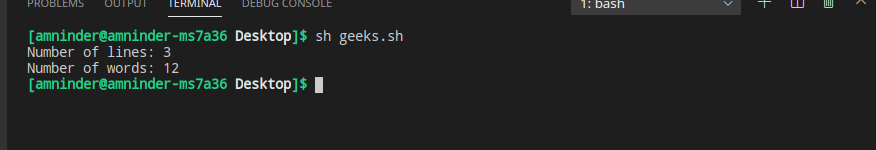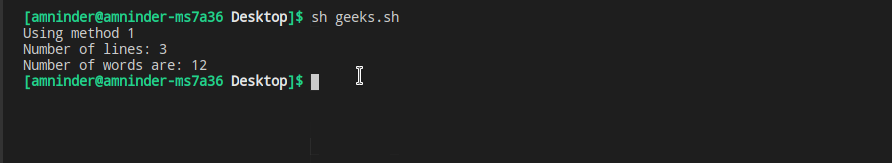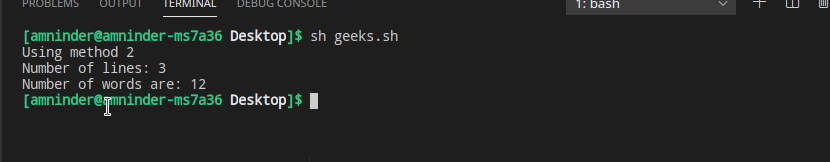Related Articles

# Shell Script to Count Lines and Words in a File

• Last Updated : 18 May, 2021

Linux provides users a great cool feature of the command-line tool along with a graphical user interface where they can perform tasks via ruining command. All of this command returns a status according to their execution. Its execution value can be used for showing errors or take some other action in a shell script.

There may be some scenarios where one needs to keep track of the number of lines and number of words in a particular file. In that scenario, any of the following methods can be used to count the number of the lines and the words in a particular file in Linux. Let’s take some examples for better understanding:

Example: Consider this file(demo.txt) with the following content:

```This is first line.
This is second line.
This is third line.```

Output:

```Number of line = 3
Number of words = 12```

Let us have a look at all the methods to count the number of lines and words and how they can be used in a shell script.

Method 1: Using WC command

wc stands for word counts. Using wc command the number of words, number of lines, number of white spaces, etc can be determined.

Syntax-

`wc [option] [input-file]`

Approach:

1. Create a variable to store the file path.
2. Use wc –lines command to count the number of lines.
3. Use wc –word command to count the number of words.
4. Print the both number of lines and the number of words using the echo command.

Input file: cat demo.txt

```This is first line
This is second line
This is third line```

cat command is used to show the content of the file.

Script:

```#!/usr/bin/bash

# path to the file
file_path="/home/amninder/Desktop/demo.txt"

# using wc command to count number of lines
number_of_lines=`wc --lines < \$file_path`

# using wc command to count number of words
number_of_words=`wc --word < \$file_path`

# Displaying number of lines and number of words
echo "Number of lines: \$number_of_lines"
echo "Number of words: \$number_of_words"```

Output:Using wc command

Explanation:

• The first line tells the system that bash will be used as an interpreter.
• The wc command is used to find out the number of lines and number of words.
• A variable is created to hold the file path.
• After that, wc command is used with –lines argument to count the number of lines, and similarly, wc command with –words argument is used to count the number of words in the file.
• In the end, the number of words and the number of lines is displayed using the echo command.

NOTE: Lines starting with the “#” symbol are called comments and ignored by the interpreter except for the first line.

Method 2: Using awk command

awk is a scripting language mainly used for text preprocessing and text manipulation. Using awk, we can do pattern search, find and replace, count words, count lines, count special symbols, count white spaces, etc.

Syntax:

`awk {action-to-be-performed} [input-file]`

Approach 1:

• Create a variable to store the file path.
• Initialize a counter variable to count the number of lines.
• After every line increment the counter variable to count the number of lines.
• Display the number of lines using the print command.
• Initialize another counter variable to count the number of words.
• Use white space as a Record Separator and increment the counter variable to count the number of words separated by space.
• After that, display the number of words using the print command.

Script:

```#!/usr/bin/bash

# path to the file
file_path="/home/amninder/Desktop/demo.txt"

# Method 1
echo "Using method 1"
# using awk command to count number of lines
awk 'BEGIN{c1=0} //{c1++} END{print "Number of lines: ",c1}' \$file_path

#using awk command to count number of words
awk 'BEGIN{c=0} //{c++} END{print "Number of words: ",c}' RS="[[:space:]]" \$file_path```

Output:Using method 1

Explanation:

1. In the first line, a variable file_path is created to hold the path of the text file.
2. The awk command statement can be divided into the following parts.
• BEGIN{c=0} will initialize a count variable called. //{c++} will increment the count variable c by 1, whenever it encountered a new line.
• END{print “Number of lines: “, c} will print the number of lines.
3. Similarly, the number of words are counted by separating each word by space using RS=”[[:space:]]. Here, RS is a Record Separator, and space is used as a separator in this example.

Note: Lines starting with the “#” symbol are called comments and ignored by the interpreter except for the first line.

Approach 2:

1. Create a variable to store the file path.
2. Use a special NR variable to find out the number of lines. NR means the number of records, and it holds the number of processed records.
3. Use NF(Number of fields in the current record) to find out the number of words in each line.
4. Then use a while loop to traverse through all the lines and sum up the NF from each line.
5. Display the number of lines.

Example for NF: Let’s file be-

```First line is on top
Second line is on second last position```

NF means the number of fields in the current record i.e. number of words in the current line.

```command:
awk '{print NF}' demo.txt

Output:
5
7```

Here, 5 represents that there are 5 words in the first line, and 7 means there are 7 words in the second line.

Script:

```#!/usr/bin/bash

# path to the file
file_path="/home/amninder/Desktop/demo.txt"

# Method 2
echo "Using method 2"

# using NR to count number of lines
awk 'END{print "Number of lines:",NR}' \$file_path

#using awk command to count number of words
awk '{i=0; count=0; while (i<NR) { count+=NF; i++;}}
END {print "Number of words are: " count}' \$file_path```

Output:Using method 2

Explanation:

• In the first line, a variable file_path is created to hold the path of the text file.
• Then, the number of lines is printed using ‘END{print “Number of lines:”,NR}’.
• Here, END represents that we are interested in the last values of the NR variable as NR variable holds the count of the processed records.
• To count the number of lines, a while loop is used till the number of processed records.
• Adding the value of the NF i.e. count of words in each line.
• In the end, the number of words is printed that is stored in the count variable.

My Personal Notes arrow_drop_up